# DFT study of the Optoelectronic properties of Sn1- xAxS (A= Au and Ag) Solar Cell Applications

1Department of Physics, The University of Lahore, Sargodha campus, 40100 Sargodha, Pakistan

2Faculty of Engineering and Applied Sciences, Department of Physics, RIPHAH International University I-14 Campus, Islamabad, Pakistan

Received Date: November 19, 2020;  Published Date: December 01, 2020

#### Abstract

Doping effect of transition metal (TM) atoms on the photovoltaic features of Sn1-xAxS (A= Au and Ag) is investigated theoretically. DFT based calculations are used to explore electronic and optical properties by using full potential linearized augmented plane wave (FP-LAPW) method. Furthermore, generalized gradient (GGA) approximation is applied for the treatment of exchange and correlation potential. Ground state properties of these compounds are calculated by using GGA approximation. Our investigation shows that these compounds are metallic in nature. Origin of atomic/orbital electronic states in the energy band dispersion are explored in detail for S, Sn, Au and Ag from the calculated spectra of density of states. Optical parameters like complex dielectric function are also evaluated by using the information of energy band dispersions along with other associated optical properties like refractive index, optical conductivity, extinction coefficient, absorption coefficient, energy loss function and reflectivity coefficient. This study confirms that these TM based compounds can be used as promising compounds for technological optoelectronic applications having desired properties.

#### Introduction

In recent years, researchers have developed their interests in intermediate band (IB) materials due to their technological application is solar cells . In next generation solar cells, the problem of efficiency can be overcome by developing IB photovoltaic materials. The working demonstration of intermediate solar cells is shown in Figure 1.Arrows in the diagram show the possible electronic transitions. These electronic transitions can also take place in traditional way from valance band (VB) to conduction band (CB). In above figure, energy gaps between IB-CB, VB-IB and VB-CB are represented by Eg3, Eg2 and Eg1, respectively, and Fermi level is specified by EF. It can also be noted that IB can offer accommodation for the electrons travelling from VB to CB with less energy as compared to Eg1. Electrons can move from VB to IB and then from IB to CB in the materi als possessing IB. Several features of solar cells based on IB materials are presented in Figure 1. Existence of an isolated IB at Fermi level is the most prominent among all feature. Thermal relaxations can be produced due to interaction of electrons and the phonons of crystal lattice if this condition is not satisfied . Moreover, IB must have a specified thickness so that the phenomenon of non-radiative recombination be avoided as much as possible. Electrons coming from VB with lower energies as compared to the energy difference between VB and CB can stay in IB only if it is partially filled.

Solar cells with this construction have higher conversion efficiencies then those established by Shockley limit , as reported earlier by Luque and Marti . They reported the increase in the efficiency of these solar cells by operation and thermodynamic arguments. Diluted oxides with II-VI composition  or quantum dots can be introduced in the geometry  to prepare materials having IB.

Main focus of this investigation is the study of optical and electronic properties of Sn1-xAxS (A= Au and Ag) having IB with the help of quantum mechanical methodology. Additionally, electronic and optical properties of aforesaid compounds can also be calculated by using this methodology. We will discuss methodology and then investigated results will be discussed in the successive parts.

#### Methodology

Kohn-Sham equations are solved in order to calculate ground state properties of Sn1-xAxS (A= Au and Ag) by using self-consistent approach with the help of full potential linearized augmented plane wave (FP-LAPW) method  within the framework of DFT [7,8] as executed in WIEN2K code [9,10]. Exchange and correlation energy (Exc) were treated by using GGA approximation while calculating optical and electronic properties of Sn1-xAxS (A= Au and Ag). Optimized geometric structure was achieved through minimization of forces from the atoms of unit cell to carry out this DFT study. Kohn-Sham equations are solved in order to calculate ground state properties of Sn1-xAxS (A= Au and Ag) by using self-consistent approach with the help of full potential linearized augmented plane wave (FP-LAPW) method  within the framework of DFT [7,8] as executed in WIEN2K code [9,10]. Exchange and correlation energy (Exc) were treated by using GGA approximation while calculating optical and electronic properties of Sn1-xAxS (A= Au and Ag). Optimized geometric structure was achieved through minimization of forces from the atoms of unit cell to carry out this DFT study.

Muffin-tin spheres and the interstitial region (IR) are the two main parts of the crystal unit cell while working with FP-LAPW method. Here, core and valance electrons are treated as two separate groups of the electrons. In this technique, muffin-tin (MT) model is used to drive crystal potential. Valance electrons and non-overlapping atomic spheres with radius RMT (smallest muffin-tin radius) are termed as IR and muffin-tin spheres. The product of Spherical harmonics (Ylm) and radial solution of Schrodinger wave equation (SWE) at fixed energy for single particle (Vlm) is used to specify potential in muffin-tin spheres.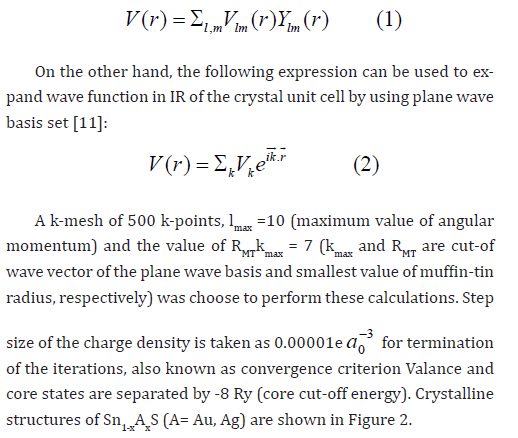#### Results and Discussion

##### Electronic properties

In this section, we have presented the electronic properties of Sn1-xAxS (A= Au, Ag) namely the energy band structures and the density of states. Energy band structures are presented along highly symmetric points of Brillouin zone for a continuous energy range of -5.0 to 5.0 eV. Density of states are presented for a continuous energy range of -6.0 to 6.0 eV to determine the possible electronic transitions from CB to VB.

##### Band structure

In this section, energy band structures for the supercells of Sn1- xAxS (A= Au, Ag) are discussed along highly symmetric points of the irreducible Brillouin zone (IBZ). Calculated structures are shown in Figure 3 by setting Fermi level at 0 eV. An entirely isolated extra band can be seen at Fermi level of both the band structures of Sn1- xAxS (A= Au, Ag) known as intermediate band (IB). We can see that this band cross Fermi level. Intermediate bands can be divided into three types depending on the number of electrons in the IB as type I, type II and type III as shown in Figure 4. Type I and III are completely empty and they can be used to store electronic charge in the form of holes and electrons . In literature, we can find several materials that carry IB for Li-ion batteries such as LiMn2O4, LiCoO2 etc . 1-xAxS (A= Au, Ag) are potential candidates for technological applications in solar cells due to their partially filled IB. Materials with type-II IB are the main focus of this study. We can observe that energy band gap is present between IB and CB. This band gap decreases as we use Au as dopant instead of Ag. Energy band gap between IB and CB is approximately 1.0 and 1.5 eV for 1-xAxS and Sn1-xAgxS, respectively.##### Density of states

Partial (PDOS) and total density states (TDOS) are calculated to get insight of the possible electronic transitions and their impact on various ground state properties. Calculated spectra of DOS for Sn1- xAxS and Sn1-xAxS are presented in Figure 5 and Figure 6, respectively. We can observe from Figure 5 that Au-atoms have key impact in the valance band of Sn1-xAxS , however, minor impact of S and Sn-atoms is also present. An IB due to hybridization of Au-d and S-p orbitals is also present around Fermi level. In conduction band, we can observe that S and Sn-atoms have key impact, however, minor impact of Au-atoms is also present.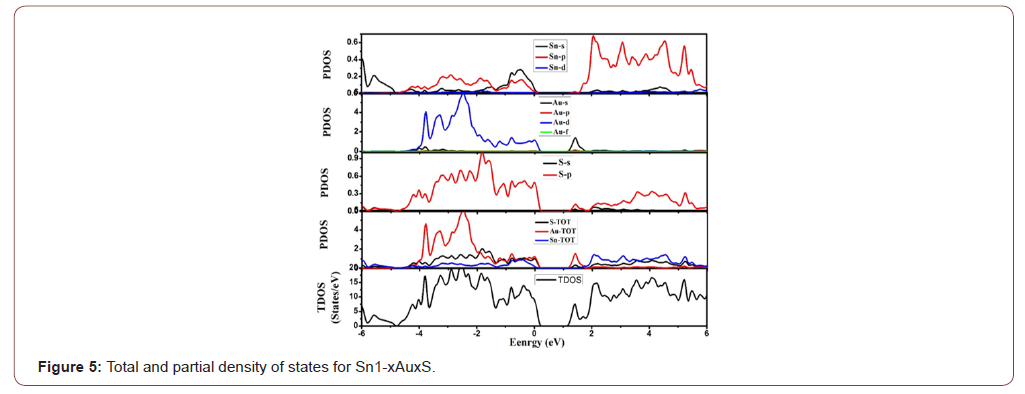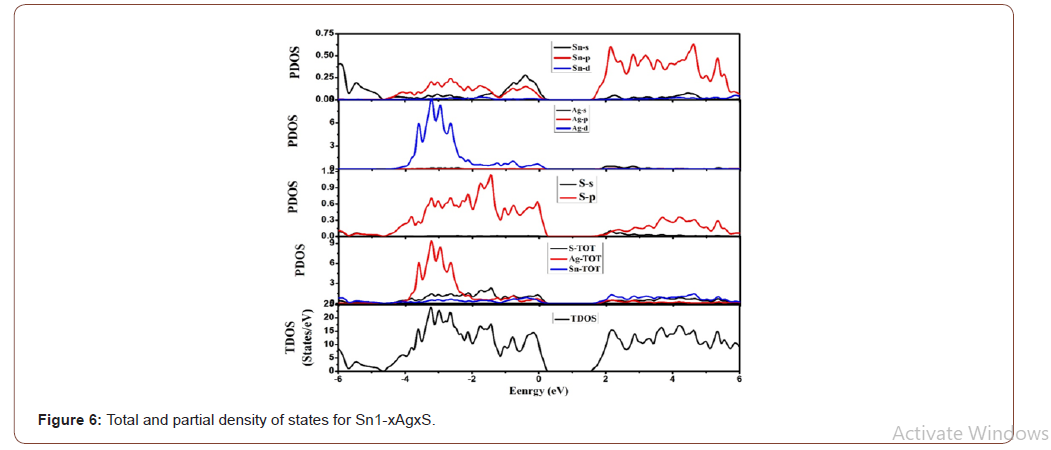From the spectra of PDOS, we can note that VB can be divided into three parts as part A (-6.0 to -4.0 eV), part B (-4.0 to -2.0 eV) and part C (-2.0 to 0 eV). In part A, possible electronic transitions occur only due Sn[5s2] orbitals because all other orbitals are silent in this region. In part B, major electronic contributions come from Sn[5p2], Au[5d10] and S[3p4]. Finally, in part C of the VB, major electronic contributions come from Sn[5p2], Sn[5s2], Au[5d10] and S[3p4]. From the spectra of PDOS, we can note that S[3p4] and Sn[5p2] orbitals have key impact in CB, however, minor impact of Au[6S1] is also present.

We can observe from Figure 6 that Ag-atoms have key impact in the central part of valance band of Sn1-xAuxS, however, minor impact of S and Sn-atoms is also present. An IB due to hybridization of Ag-d and S-p orbital is also present around Fermi level. In conduction band, we can observe that S and Sn-atoms have key impact, however, minor impact of Ag-atoms is also present [12,13].

From the spectra of PDOS, we can note that VB can be divided into three parts as part A (-6.0 to -4.0 eV), part B (-4.0 to -2.0 eV) and part C (-2.0 to 0 eV). In part A, possible electronic transitions occur only due Sn[5s2] orbitals because all other orbitals are silent in this region. In part B, major electronic contributions come from Sn[5p2], Ag[4d10] and S[3p4]. Finally, in part C of the VB, major electronic contributions come from Sn[5p2], Sn[5s2], Ag[4d10] and S[3p4 ]. From the spectra of PDOS, we can note that S[3p4] and Sn[5p2] orbitals have key impact in CB, however, minor impact of Ag[5S1] is also present.

##### Fermi surface

Due to metallic nature of Sn1-xAxS (A= Au, Ag), Fermi surfaces (FS) are also investigated for the accessible electronic states of these compounds. Configuration of near Fermi bands present in Sn1-xAxS (A= Au, Ag) are shown in Figure 7 for the highly symmetric points of IBZ. Electrons near Fermi level play an important role in the electronic conductivity of the material. Understanding of the electronic properties of metallic compounds (band structure and DOS) can be obtained from their FS. Comprehensive knowledge of the electronic states (at Fermi level (EF)) can be obtained from FS. Electronic sheets and holes in the FS are represented by colored region and the empty spaces, respectively. Maximum region of the FS is empty for both compounds which show that these compounds are p-type semiconductors. From band structure, we can note that there is only one band that cross EF in both compounds. Thermoelectric properties such as electrical conductivity is directly related to the topography of the FS. Electrons with various velocities are represented by different colors in the FS.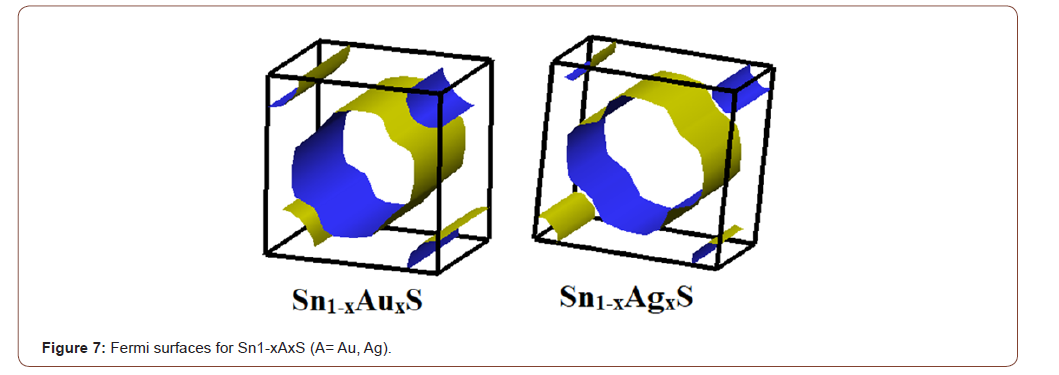##### Optical properties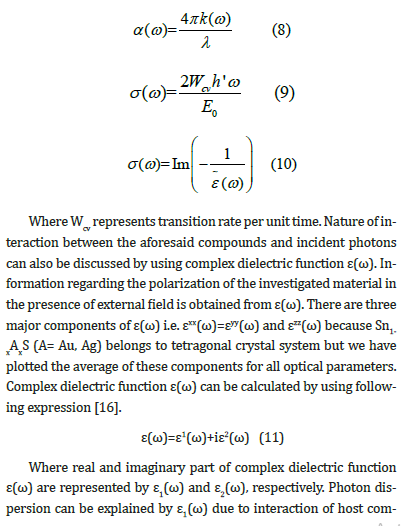pound and the incident photons. It is used to discuss interbond electronic transitions due to the absorption of incident photons. Kramer-Kronig expression can be used to associate these parameters with other optical parameters [17,18]. All optical parameters are calculated over a continuous energy range of 0 to 14 eV.

Real part ε1 (ω) of complex dielectric function over an entire energy range of 0 to 14 eV is shown in Figure 8(a). Major peaks of ε1 (ω) are present in visible and infrared region. After 2.5 eV, curves for both materials start decreasing and enters the negative region at approximately 5.5 eV. These negative values of ε1(ω) corresponds to the metal like reflecting nature of 1-xAxS (A= Au, Ag). Zero frequency limit (static values) of ε1(0) can also be determined with the help of calculated spectra of ε1(ω). Estimated values of ε1(0) are appeared to be 14.3 and 14.2 for 1-xAxS and 1-xAxS, respectively. Energy band gap Eg and ε1(0) are inversely related to each other. Following expression of the Penn’s model can be used to relate Eg and ε1(0) .Refractive index n(ω) and extinction coefficient k(ω) calculated for 1-xAxS (A= Au, Ag) are shown in Figure 8(c) and 8(d), respectively, over an entire energy range of 0 to 14 eV. Calculated values of ε1 (ω) and ε2 (ω) can be used to calculate n(ω) and k(ω) with the help of following expressions:Refractive index n(ω) can be used to discuss transparency and dispersion behavior of the compounds. Major peaks of n(ω) are present in visible and infrared region. After 2.5 eV, curves for both materials start decreasing and becomes less that unity at approximately 7.0 eV. It shows that photons with these energies are opposed by the polarization of aforesaid compounds because speed of light become less than the group velocity (Vg=c/n) . Zero frequency limit (static values) of n(0) can also be determined with the help of calculated spectra of n(ω). Estimated values of n(0) are appear to be 3.79 for both 1-xAxS and 1-xAxS. Energy band gap Eg and ε1 (0) are inversely related to each other. Following expression of the Penn’s model can be used to relate n(0) and ε1 (0).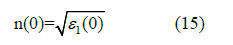Extinction coefficient k(ω) can be used to explain the features similar to that of ε2 (ω) like absorption of light. Major peaks of k(ω) are present in ultraviolet region of the optical spectrum. In the spectra of k(ω), a unique property is its critical value. No transmission of photons is present in 1-xAxS (A= Au, Ag) as their threshold energy is below 0 eV, so, all the incident photons are absorbed by these materials. Prominent peaks are present at approximately 0.5 eV and then at approximately 6.0 eV for both compounds in the spectra of k(ω). Some peaks are also present in visible region between 1.7 and 3.1 eV.

Reflectivity R(ω) and energy loss function L(ω) calculated for Sn1-xAxS (A= Au, Ag) are shown in Figure 9(a) and 9(b), respectively, over an entire energy range of 0 to 14 eV. Surface study of 1-xAx (A= Au, Ag) is also discussed by calculating the difference of the reflected power and incident power known as reflectivity R(ω). Zero frequency limit (static values) of R(0) can also be determined with the help of calculated spectra of R(ω). Estimated values of R(0) are appear to be 0.341 and 0.342 both 1-xAx and 1-xAx, respectively. Behavior of R(ω) and ε1 (ω) is similar to each other. Hence, R(ω) and absorption coefficient I(ω) are inversely related to each other which implies that I(ω) will be lower in the region where the values of R(ω) are higher and vice versa. Various peaks are present in the spectra of R(ω) because of anisotropy of the material. However, R(ω) show its maximum peak in UV region where ε1 (ω) is negligible because in this region energy gap is zero and material reflects almost all the incident photons due to its reflecting metallic nature.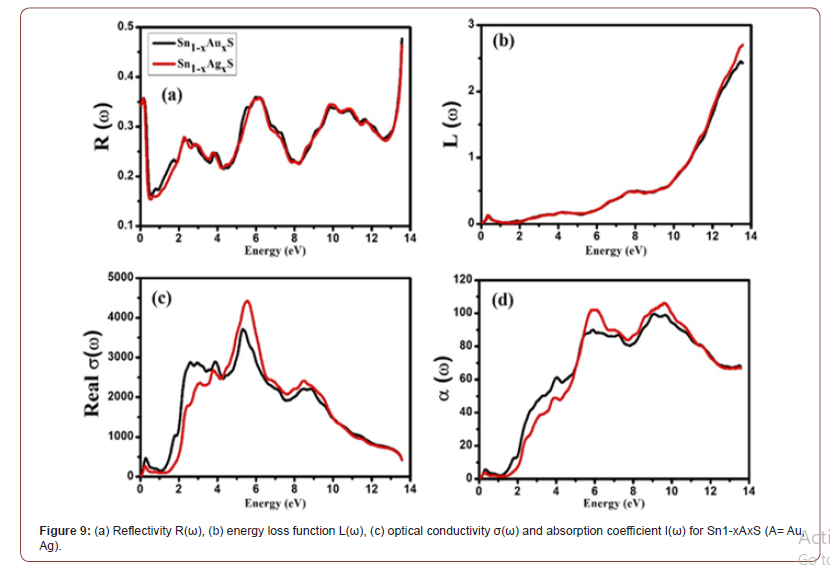Loss of rapidly moving electrons in the material can be explained by an important optical parameter known as energy loss function L(ω). Plasma oscillations are responsible for the characteristic behavior of the peaks present in the spectra of L(ω) and the frequencies associated with these oscillations are known as plasma frequencies. Plasmon excitations are responsible for the major peaks in the calculated spectra of L(ω). It is due to the mutual oscillations of the background atomic cores against the valance electrons in the longitudinal fashion with plasma frequency ωp. We can note from the spectra of L(ω) that there is negligible loss of electron energy up to 10.0 eV and then the spectra of L(ω) becomes maximum in upper UV region [17-20].

Optical conductivity σ(ω) and absorption coefficient α(ω) calculated for Sn1-xAxS (A= Au, Ag) are shown in Figure 9(c) and 9(d), respectively, over an entire energy range of 0 to 14 eV. Measure of the produced charge carriers due to breaking of the bond of atoms in the material due to incident photons which gives reasonable forward current is presented by σ(ω). Highest peaks of σ(ω) are present between 2.0 to 8.0 eV. We can note from the variations of σ(ω) show similar behavior as that of α(ω), k(ω) and ε2 (ω). Absorption of incident photons by the host materials is responsible for this behavior of σ(ω).

Information regarding penetration length of the incident photons inside the host material is given by absorption coefficient α(ω). Absorption coefficient α(ω) can be calculated by using the following expression :Both materials show no absorption of incident photons till 1.5 eV, inside the outlawed energy band. Because of electronic transitions from VB to CB, we can note prominent peaks in the spectra of α(ω) between 5.0 to 11.0 eV.

#### Conclusion

GGA potential scheme is used with DFT based FP-LAPW approach to explore the effect of TM atoms on ground state properties of Sn1-xAxS (A= Au, Ag). Electronic properties show that these compounds are metallic in nature. GGA functional is employed to calculate band structures and DOS (total and partial) in order to get accurate knowledge of the electronic properties. Our calculations show that these compounds possess intermediate band and energy band gap (between IB and CB) of these materials decreases as we change dopant from Ag to Au. We conclude that these materials are p-type semiconductors above IB. Optical parameters for Sn1-xAxS (A= Au, Ag) are also calculated to get insights of the usability of these compounds in technological optical applications. We conclude that these materials are not good reflectors as they reflect maximum of 50 % of the incident photons in upper UV region.

#### Acknowledgement

The work is supported by the Higher Education Commission of Pakistan start-up research grants No: 21-2344/SRGP/R&D/ HEC/2019 and 21-2340/SRGP/R&D/HEC/2019.

#### Conflict of Interest

No conflict of interest.

Article Details
Citation
Keywords
Scroll to
Scroll to Top